## Download PDF

How to read this lecture...

Code should execute sequentially if run in a Jupyter notebook

# Optimal Growth II: Time Iteration¶

## Contents¶

In addition to what’s in Anaconda, this lecture will need the following libraries:

In :
!pip install --upgrade quantecon
!pip install interpolation


## Overview¶

In this lecture, we’ll continue our earlier study of the stochastic optimal growth model.

In that lecture, we solved the associated discounted dynamic programming problem using value function iteration.

The beauty of this technique is its broad applicability.

With numerical problems, however, we can often attain higher efficiency in specific applications by deriving methods that are carefully tailored to the application at hand.

The stochastic optimal growth model has plenty of structure to exploit for this purpose, especially when we adopt some concavity and smoothness assumptions over primitives.

We’ll use this structure to obtain an Euler equation based method that’s more efficient than value function iteration for this and some other closely related applications.

In a subsequent lecture, we’ll see that the numerical implementation part of the Euler equation method can be further adjusted to obtain even more efficiency.

Let’s start with some imports

In :
import numpy as np
import quantecon as qe
from interpolation import interp
from numba import njit, prange
from quantecon.optimize import brentq
from quantecon.optimize.scalar_maximization import brent_max
import matplotlib.pyplot as plt
%matplotlib inline


## The Euler Equation¶

Let’s take the model set out in the stochastic growth model lecture and add the assumptions that

1. $u$ and $f$ are continuously differentiable and strictly concave
2. $f(0) = 0$
3. $\lim_{c \to 0} u'(c) = \infty$ and $\lim_{c \to \infty} u'(c) = 0$
4. $\lim_{k \to 0} f'(k) = \infty$ and $\lim_{k \to \infty} f'(k) = 0$

The last two conditions are usually called Inada conditions.

Recall the Bellman equation

$$v^*(y) = \max_{0 \leq c \leq y} \left\{ u(c) + \beta \int v^*(f(y - c) z) \phi(dz) \right\} \quad \text{for all} \quad y \in \mathbb R_+ \tag{1}$$

Let the optimal consumption policy be denoted by $\sigma^*$.

We know that $\sigma^*$ is a $v^*$ greedy policy so that $\sigma^*(y)$ is the maximizer in (1).

The conditions above imply that

• $\sigma^*$ is the unique optimal policy for the stochastic optimal growth model
• the optimal policy is continuous, strictly increasing and also interior, in the sense that $0 < \sigma^*(y) < y$ for all strictly positive $y$, and
• the value function is strictly concave and continuously differentiable, with

$$(v^*)'(y) = u' (\sigma^*(y) ) := (u' \circ \sigma^*)(y) \tag{2}$$

The last result is called the envelope condition due to its relationship with the envelope theorem.

To see why (2) might be valid, write the Bellman equation in the equivalent form

$$v^*(y) = \max_{0 \leq k \leq y} \left\{ u(y-k) + \beta \int v^*(f(k) z) \phi(dz) \right\},$$

differentiate naively with respect to $y$, and then evaluate at the optimum.

Section 12.1 of EDTC contains full proofs of these results, and closely related discussions can be found in many other texts.

Differentiability of the value function and interiority of the optimal policy imply that optimal consumption satisfies the first order condition associated with (1), which is

$$u'(\sigma^*(y)) = \beta \int (v^*)'(f(y - \sigma^*(y)) z) f'(y - \sigma^*(y)) z \phi(dz) \tag{3}$$

Combining (2) and the first-order condition (3) gives the famous Euler equation

$$(u'\circ \sigma^*)(y) = \beta \int (u'\circ \sigma^*)(f(y - \sigma^*(y)) z) f'(y - \sigma^*(y)) z \phi(dz) \tag{4}$$

We can think of the Euler equation as a functional equation

$$(u'\circ \sigma)(y) = \beta \int (u'\circ \sigma)(f(y - \sigma(y)) z) f'(y - \sigma(y)) z \phi(dz) \tag{5}$$

over interior consumption policies $\sigma$, one solution of which is the optimal policy $\sigma^*$.

Our aim is to solve the functional equation (5) and hence obtain $\sigma^*$.

### The Coleman-Reffett Operator¶

Recall the Bellman operator

$$Tw(y) := \max_{0 \leq c \leq y} \left\{ u(c) + \beta \int w(f(y - c) z) \phi(dz) \right\} \tag{6}$$

Just as we introduced the Bellman operator to solve the Bellman equation, we will now introduce an operator over policies to help us solve the Euler equation.

This operator $K$ will act on the set of all $\sigma \in \Sigma$ that are continuous, strictly increasing and interior (i.e., $0 < \sigma(y) < y$ for all strictly positive $y$).

Henceforth we denote this set of policies by $\mathscr P$

1. The operator $K$ takes as its argument a $\sigma \in \mathscr P$ and
2. returns a new function $K\sigma$, where $K\sigma(y)$ is the $c \in (0, y)$ that solves.

$$u'(c) = \beta \int (u' \circ \sigma) (f(y - c) z ) f'(y - c) z \phi(dz) \tag{7}$$

We call this operator the Coleman-Reffett operator to acknowledge the work of [Col90] and [Ref96].

In essence, $K\sigma$ is the consumption policy that the Euler equation tells you to choose today when your future consumption policy is $\sigma$.

The important thing to note about $K$ is that, by construction, its fixed points coincide with solutions to the functional equation (5).

In particular, the optimal policy $\sigma^*$ is a fixed point.

Indeed, for fixed $y$, the value $K\sigma^*(y)$ is the $c$ that solves

$$u'(c) = \beta \int (u' \circ \sigma^*) (f(y - c) z ) f'(y - c) z \phi(dz)$$

In view of the Euler equation, this is exactly $\sigma^*(y)$.

### Is the Coleman-Reffett Operator Well Defined?¶

In particular, is there always a unique $c \in (0, y)$ that solves (7)?

The answer is yes, under our assumptions.

For any $\sigma \in \mathscr P$, the right side of (7)

• is continuous and strictly increasing in $c$ on $(0, y)$
• diverges to $+\infty$ as $c \uparrow y$

The left side of (7)

• is continuous and strictly decreasing in $c$ on $(0, y)$
• diverges to $+\infty$ as $c \downarrow 0$

Sketching these curves and using the information above will convince you that they cross exactly once as $c$ ranges over $(0, y)$.

With a bit more analysis, one can show in addition that $K \sigma \in \mathscr P$ whenever $\sigma \in \mathscr P$.

## Comparison with Value Function Iteration¶

How does Euler equation time iteration compare with value function iteration?

Both can be used to compute the optimal policy, but is one faster or more accurate?

There are two parts to this story.

First, on a theoretical level, the two methods are essentially isomorphic.

In particular, they converge at the same rate.

We’ll prove this in just a moment.

The other side of the story is the accuracy of the numerical implementation.

It turns out that, once we actually implement these two routines, time iteration is more accurate than value function iteration.

More on this below.

### Equivalent Dynamics¶

Let’s talk about the theory first.

To explain the connection between the two algorithms, it helps to understand the notion of equivalent dynamics.

(This concept is very helpful in many other contexts as well)

Suppose that we have a function $g \colon X \to X$ where $X$ is a given set.

The pair $(X, g)$ is sometimes called a dynamical system and we associate it with trajectories of the form

$$x_{t+1} = g(x_t), \qquad x_0 \text{ given}$$

Equivalently, $x_t = g^t(x_0)$, where $g$ is the $t$-th composition of $g$ with itself.

Here’s the pictureNow let another function $h \colon Y \to Y$ where $Y$ is another set.

Suppose further that

• there exists a bijection $\tau$ from $X$ to $Y$
• the two functions commute under $\tau$, which is to say that $\tau(g(x)) = h (\tau(x))$ for all $x \in X$

The last statement can be written more simply as

$$\tau \circ g = h \circ \tau$$

or, by applying $\tau^{-1}$ to both sides

$$g = \tau^{-1} \circ h \circ \tau \tag{8}$$

Here’s a commutative diagram that illustratesHere’s a similar figure that traces out the action of the maps on a point $x \in X$Now, it’s easy to check from (8) that $g^2 = \tau^{-1} \circ h^2 \circ \tau$ holds.

In fact, if you like proofs by induction, you won’t have trouble showing that

$$g^n = \tau^{-1} \circ h^n \circ \tau$$

is valid for all $n$.

What does this tell us?

It tells us that the following are equivalent

• iterate $n$ times with $g$, starting at $x$
• shift $x$ to $Y$ using $\tau$, iterate $n$ times with $h$ starting at $\tau(x)$ and shift the result $h^n(\tau(x))$ back to $X$ using $\tau^{-1}$

We end up with exactly the same object.

### Back to Economics¶

Have you guessed where this is leading?

What we’re going to show now is that the operators $T$ and $K$ commute under a certain bijection.

The implication is that they have exactly the same rate of convergence.

To make life a little easier, we’ll assume in the following analysis (although not always in our applications) that $u(0) = 0$.

#### A Bijection¶

Let $\mathscr V$ be all strictly concave, continuously differentiable functions $v$ mapping $\mathbb R_+$ to itself and satisfying $v(0) = 0$ and $v'(y) > u'(y)$ for all positive $y$.

For $v \in \mathscr V$ let

$$M v := h \circ v' \qquad \text{where } h := (u')^{-1}$$

Although we omit details, $\sigma := M v$ is actually the unique $v$-greedy policy.

• See proposition 12.1.18 of EDTC.

It turns out that $M$ is a bijection from $\mathscr V$ to $\mathscr P$.

A (solved) exercise below asks you to confirm this.

#### Commutative Operators¶

It is an additional solved exercise (see below) to show that $T$ and $K$ commute under $M$, in the sense that

$$M \circ T = K \circ M \tag{9}$$

In view of the preceding discussion, this implies that

$$T^n = M^{-1} \circ K^n \circ M$$

Hence, $T$ and $K$ converge at exactly the same rate!

## Implementation¶

We’ve just shown that the operators $T$ and $K$ have the same rate of convergence.

However, it turns out that, once numerical approximation is taken into account, significant differences arise.

In particular, the image of policy functions under $K$ can be calculated faster and with greater accuracy than the image of value functions under $T$.

Our intuition for this result is that

• the Coleman-Reffett operator exploits more information because it uses first order and envelope conditions
• policy functions generally have less curvature than value functions, and hence admit more accurate approximations based on grid point information

First, we’ll store the parameters of the model in a class OptimalGrowthModel

In :
class OptimalGrowthModel:

def __init__(self,
f,
f_prime,
u,
u_prime,
β=0.96,
μ=0,
s=0.1,
grid_max=4,
grid_size=200,
shock_size=250):

self.β, self.μ, self.s = β, μ, s
self.f, self.u = f, u
self.f_prime, self.u_prime = f_prime, u_prime

self.grid = np.linspace(1e-5, grid_max, grid_size)         # Set up grid
self.shocks = np.exp(μ + s * np.random.randn(shock_size))  # Store shocks


Here’s some code that returns the Coleman-Reffett operator, $K$.

In :
def time_operator_factory(og, parallel_flag=True):
"""
A function factory for building the Coleman-Reffett operator.
Here og is an instance of OptimalGrowthModel.
"""
β = og.β
f, u = og.f, og.u
f_prime, u_prime = og.f_prime, og.u_prime
grid, shocks = og.grid, og.shocks

@njit
def objective(c, σ, y):
"""
The right hand side of the operator
"""
# First turn w into a function via interpolation
σ_func = lambda x: interp(grid, σ, x)
vals = u_prime(σ_func(f(y - c) * shocks)) * f_prime(y - c) * shocks
return u_prime(c) - β * np.mean(vals)

@njit(parallel=parallel_flag)
def K(σ):
"""
The Coleman-Reffett operator
"""
σ_new = np.empty_like(σ)
for i in prange(len(grid)):
y = grid[i]
# Solve for optimal c at y
c_star = brentq(objective, 1e-10, y-1e-10, args=(σ, y))
σ_new[i] = c_star

return σ_new

return K


It has some similarities to the code for the Bellman operator in our optimal growth lecture.

For example, it evaluates integrals by Monte Carlo and approximates functions using linear interpolation.

Here’s that Bellman operator code again, which needs to be executed because we’ll use it in some tests below.

In :
def operator_factory(og, parallel_flag=True):
"""
A function factory for building the Bellman operator, as well as
a function that computes greedy policies.

Here og is an instance of OptimalGrowthModel.
"""

f, u, β = og.f, og.u, og.β
grid, shocks = og.grid, og.shocks

@njit
def objective(c, v, y):
"""
The right-hand side of the Bellman equation
"""
# First turn v into a function via interpolation
v_func = lambda x: interp(grid, v, x)
return u(c) + β * np.mean(v_func(f(y - c) * shocks))

@njit(parallel=parallel_flag)
def T(v):
"""
The Bellman operator
"""
v_new = np.empty_like(v)
for i in prange(len(grid)):
y = grid[i]
# Solve for optimal v at y
v_max = brent_max(objective, 1e-10, y, args=(v, y))
v_new[i] = v_max
return v_new

@njit
def get_greedy(v):
"""
Computes the v-greedy policy of a given function v
"""
σ = np.empty_like(v)
for i in range(len(grid)):
y = grid[i]
# Solve for optimal c at y
c_max = brent_max(objective, 1e-10, y, args=(v, y))
σ[i] = c_max
return σ

return T, get_greedy


### Testing on the Log / Cobb–Douglas Case¶

As we did for value function iteration, let’s start by testing our method in the presence of a model that does have an analytical solution.

First, we generate an instance of OptimalGrowthModel and return the corresponding Coleman-Reffett operator.

In :
α = 0.3

@njit
def f(k):
"Deterministic part of production function"
return k**α

@njit
def f_prime(k):
return α * k**(α - 1)

og = OptimalGrowthModel(f=f, f_prime=f_prime,
u=np.log, u_prime=njit(lambda x: 1/x))

K = time_operator_factory(og)


As a preliminary test, let’s see if $K \sigma^* = \sigma^*$, as implied by the theory.

In :
@njit
def σ_star(y, α, β):
"True optimal policy"
return (1 - α * β) * y

grid, β = og.grid, og.β
σ_star_new = K(σ_star(grid, α, β))

fig, ax = plt.subplots()
ax.plot(grid, σ_star(grid, α, β), label="optimal policy $\sigma^*$")
ax.plot(grid, σ_star_new, label="$K\sigma^*$")

ax.legend()
plt.show()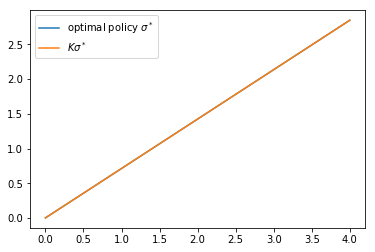We can’t really distinguish the two plots, so we are looking good, at least for this test.

Next, let’s try iterating from an arbitrary initial condition and see if we converge towards $\sigma^*$.

The initial condition we’ll use is the one that eats the whole pie: $\sigma(y) = y$.

In :
n = 15
σ = grid.copy()  # Set initial condition
fig, ax = plt.subplots(figsize=(9, 6))
lb = 'initial condition $\sigma(y) = y$'
ax.plot(grid, σ, color=plt.cm.jet(0), alpha=0.6, label=lb)

for i in range(n):
σ = K(σ)
ax.plot(grid, σ, color=plt.cm.jet(i / n), alpha=0.6)

lb = 'true policy function $\sigma^*$'
ax.plot(grid, σ_star(grid, α, β), 'k-', alpha=0.8, label=lb)
ax.legend()

plt.show()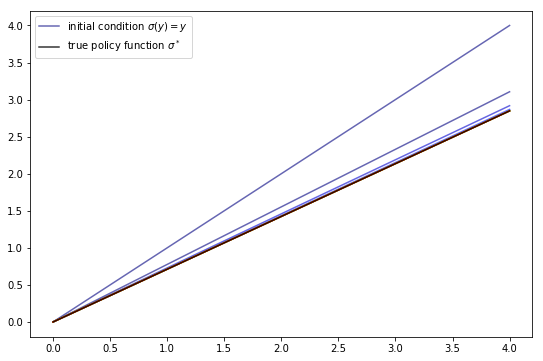We see that the policy has converged nicely, in only a few steps.

Now let’s compare the accuracy of iteration between the operators.

We’ll generate

1. $K^n \sigma$ where $\sigma(y) = y$
2. $(M \circ T^n \circ M^{-1}) \sigma$ where $\sigma(y) = y$

In each case, we’ll compare the resulting policy to $\sigma^*$.

The theory on equivalent dynamics says we will get the same policy function and hence the same errors.

But in fact we expect the first method to be more accurate for reasons discussed above

In :
T, get_greedy = operator_factory(og)  # Return the Bellman operator

σ = grid          # Set initial condition for σ
v = og.u(grid)    # Set initial condition for v
sim_length = 20

for i in range(sim_length):
σ = K(σ)  # Time iteration
v = T(v)  # Value function iteration

# Calculate difference with actual solution
σ_error = σ_star(grid, α, β) - σ
v_error = σ_star(grid, α, β) - get_greedy(v)

plt.plot(grid, σ_error, alpha=0.6, label="policy iteration error")
plt.plot(grid, v_error, alpha=0.6, label="value iteration error")
plt.legend()
plt.show()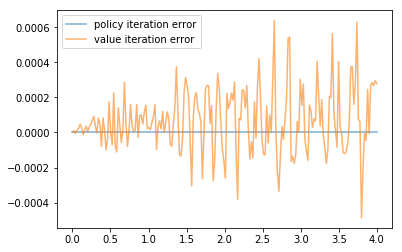As you can see, time iteration is much more accurate for a given number of iterations.

## Exercises¶

### Exercise 1¶

Show that (9) is valid. In particular,

• Let $v$ be strictly concave and continuously differentiable on $(0, \infty)$.
• Fix $y \in (0, \infty)$ and show that $MTv(y) = KMv(y)$.

### Exercise 2¶

Show that $M$ is a bijection from $\mathscr V$ to $\mathscr P$.

### Exercise 3¶

Consider the same model as above but with the CRRA utility function

$$u(c) = \frac{c^{1 - \gamma} - 1}{1 - \gamma}$$

Iterate 20 times with Bellman iteration and Euler equation time iteration

• start time iteration from $\sigma(y) = y$
• start value function iteration from $v(y) = u(y)$
• set $\gamma = 1.5$

Compare the resulting policies and check that they are close.

### Exercise 4¶

Solve the above model as we did in the previous lecture using the operators $T$ and $K$, and check the solutions are similiar by plotting.

## Solutions¶

### Exercise 1¶

Let $T, K, M, v$ and $y$ be as stated in the exercise.

Using the envelope theorem, one can show that $(Tv)'(y) = u'(\sigma(y))$ where $\sigma(y)$ solves

$$u'(\sigma(y)) = \beta \int v' (f(y - \sigma(y)) z ) f'(y - \sigma(y)) z \phi(dz) \tag{10}$$

Hence $MTv(y) = (u')^{-1} (u'(\sigma(y))) = \sigma(y)$.

On the other hand, $KMv(y)$ is the $\sigma(y)$ that solves

\begin{aligned} u'(\sigma(y)) & = \beta \int (u' \circ (Mv)) (f(y - \sigma(y)) z ) f'(y - \sigma(y)) z \phi(dz) \\ & = \beta \int (u' \circ ((u')^{-1} \circ v')) (f(y - \sigma(y)) z ) f'(y - \sigma(y)) z \phi(dz) \\ & = \beta \int v'(f(y - \sigma(y)) z ) f'(y - \sigma(y)) z \phi(dz) \end{aligned}

We see that $\sigma(y)$ is the same in each case.

### Exercise 2¶

We need to show that $M$ is a bijection from $\mathscr V$ to $\mathscr P$.

To see this, first observe that, in view of our assumptions above, $u'$ is a strictly decreasing continuous bijection from $(0,\infty)$ to itself.

It follows that $h$ has the same properties.

Moreover, for fixed $v \in \mathscr V$, the derivative $v'$ is a continuous, strictly decreasing function.

Hence, for fixed $v \in \mathscr V$, the map $M v = h \circ v'$ is strictly increasing and continuous, taking values in $(0, \infty)$.

Moreover, interiority holds because $v'$ strictly dominates $u'$, implying that

$$(M v)(y) = h(v'(y)) < h(u'(y)) = y$$

In particular, $\sigma(y) := (Mv)(y)$ is an element of $\mathscr P$.

To see that each $\sigma \in \mathscr P$ has a preimage $v \in \mathscr V$ with $Mv = \sigma$, fix any $\sigma \in \mathscr P$.

Let $v(y) := \int_0^y u'(\sigma(x)) dx$ with $v(0) = 0$.

With a small amount of effort, you will be able to show that $v \in \mathscr V$ and $Mv = \sigma$.

It’s also true that $M$ is one-to-one on $\mathscr V$.

To see this, suppose that $v$ and $w$ are elements of $\mathscr V$ satisfying $Mv = Mw$.

Then $v(0) = w(0) = 0$ and $v' = w'$ on $(0, \infty)$.

The fundamental theorem of calculus then implies that $v = w$ on $\mathbb R_+$.

### Exercise 3¶

Here’s the code, which will execute if you’ve run all the code above

In :
γ = 1.5   # Preference parameter

@njit
def u(c):
return (c**(1 - γ) - 1) / (1 - γ)

@njit
def u_prime(c):
return c**(-γ)

og = OptimalGrowthModel(f=f, f_prime=f_prime, u=u, u_prime=u_prime)

T, get_greedy = operator_factory(og)
K = time_operator_factory(og)

σ = grid        # Initial condition for σ
v = u(grid)     # Initial condition for v
sim_length = 20

for i in range(sim_length):
σ = K(σ)  # Time iteration
v = T(v)  # Value function iteration

plt.plot(grid, σ, alpha=0.6, label="policy iteration")
plt.plot(grid, get_greedy(v), alpha=0.6, label="value iteration")
plt.legend()
plt.show()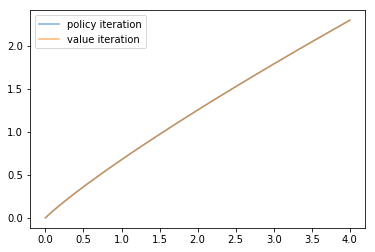The policies are indeed close.

### Exercise 4¶

Here’s is the function we need to solve the model using value function iteration, copied from the previous lecture

In :
def solve_model(og,
use_parallel=True,
tol=1e-4,
max_iter=1000,
verbose=True,
print_skip=25):

T, _ = operator_factory(og, parallel_flag=use_parallel)

# Set up loop
v = np.log(og.grid)  # Initial condition
i = 0
error = tol + 1

while i < max_iter and error > tol:
v_new = T(v)
error = np.max(np.abs(v - v_new))
i += 1
if verbose and i % print_skip == 0:
print(f"Error at iteration {i} is {error}.")
v = v_new

if i == max_iter:
print("Failed to converge!")

if verbose and i < max_iter:
print(f"\nConverged in {i} iterations.")

return v_new


Similarly, we can write a function that uses K to solve the model.

In :
def solve_model_time(og,
use_parallel=True,
tol=1e-4,
max_iter=1000,
verbose=True,
print_skip=25):

K = time_operator_factory(og, parallel_flag=use_parallel)

# Set up loop
σ = og.grid  # Initial condition
i = 0
error = tol + 1

while i < max_iter and error > tol:
σ_new = K(σ)
error = np.max(np.abs(σ - σ_new))
i += 1
if verbose and i % print_skip == 0:
print(f"Error at iteration {i} is {error}.")
σ = σ_new

if i == max_iter:
print("Failed to converge!")

if verbose and i < max_iter:
print(f"\nConverged in {i} iterations.")

return σ_new


Solving both models and plotting

In :
v_star = solve_model(og)
σ_star = solve_model_time(og)

plt.plot(grid, get_greedy(v_star), alpha=0.6, label='Bellman operator')
plt.plot(grid, σ_star, alpha=0.6, label='Coleman-Reffett operator')
plt.legend()
plt.show()

Error at iteration 25 is 0.4048676330444323.
Error at iteration 50 is 0.1459129656717657.
Error at iteration 75 is 0.05258655377547328.
Error at iteration 100 is 0.01895202133147933.
Error at iteration 125 is 0.006830246265749906.
Error at iteration 150 is 0.002461598329624337.
Error at iteration 175 is 0.0008871519564443986.
Error at iteration 200 is 0.0003197266524850306.
Error at iteration 225 is 0.0001152284360337319.

Converged in 229 iterations.

Converged in 10 iterations.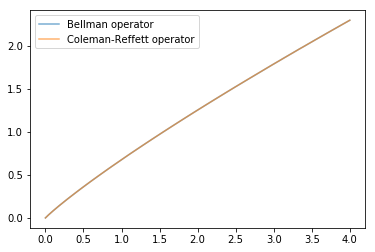Time iteration is numerically far more accurate for a given number of iterations.

• Share page